Synergetics. Etudes 70.

Is Dedicated to Centennial of Burthday of Outstanding Scientist, Professor

Basin Abram M.

Basina G. I., Basin M. A.

SIC ”Synergetics” of Saint-Petersburg Association of Scientist and Scholars.

Etude 3.

Exponent of Circumference as the Phase Trajectory of Nonlinear Iteration Relation.

2 December 2009

In this etude are determined criteria of equivalence of complex differential equation of first order and nonlinear iteration. Conditions, at which the points, corresponding to the decision of iterative relation, lay on the exponent of circumference, are determined.

At the investigation of dynamically systems may be introduced the complex parameter of wholeness, found and solved complex usual differential equation, to which it satisfies: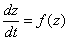.                                                    (1)

Here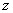may be complex algebraic variable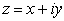or complex spiral variable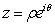. Family of phase trajectories, which are the aggregate of decisions of equation (1), may be represented in the form: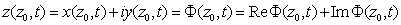,                  (2)

where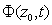- the meaning of complex coordinate of phase trajectory passing  through the pointat the moment of time 0. This decision may be written and in spiral variables: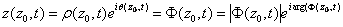.                        (3)

Shall we build iteration relation equivalent to the described dynamically systemе. Shall we solve equations (2, 3) to the relation of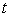: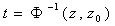.                                                 (4)

Suppose, that we know the condition of one-dimensional complex dynamically system in moment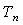, corresponding to the point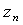and want to determine the condition of the same system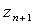at the moment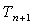. Then, using former formulas, we shall receive: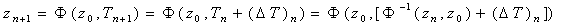.          (5)

We shall introduce the operator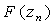: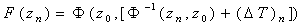.                                 (6)

Operatorgenerates the iteration process and shows transformation of condition of dynamically systemat the moment of timein its condition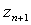at the moment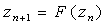.                                                        (7)

The last equation describes discrete system, equivalent to the continuous dynamically system.

It is another method of undergoing from continuous model to the discrete model. Shall we write instead of system (1) the approximate system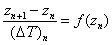,                                               (8)

which, after series of transformations, becomes the form: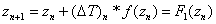.                               (9)

Operatorsand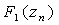are not equivalent: one aspirates to another at the attention ofto zero. Shall we consider one practically important particular case. As the base equation shall we take linear complex equation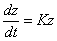.                                                                    (10)

Decision of this equation has the form: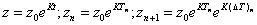.                                    (11)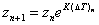.                                                      (12).

So, in the case of constant time interval, the iterative process of the first type, satisfying the equalities (5-7), represents geometric series at the area of complex numbers. If the value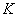is imaginary, then all members of series (12) lay on the circumference with radius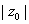.

Iterative process (9) in our particular case becomes the form: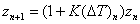.                                                (13)

Formulas (12) and (13) coincide only in limiting case at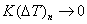.

In the case of finite meanings of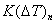these iterative processes distinguish one from another then more as greater is the modulus of value. But in the second case we also have the geometric series. Shall we introduce in consideration new complex variable: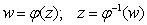.                                              (14)

Then the equation (1) transforms in the form: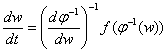,-                                             (15)

and corresponding iterative processes have the next form: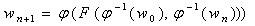.                                   (16)

Last equation describes iterative process, which is equivalent to the equation (15).

Another, approximate variant of iterative process, corresponding to the equation (15), has the form: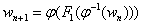.                                                     (17)

In considered earlier particular case of linear differential equation shall we take, that the relation of new variable from old variable has the form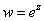.

Then the iterative relation (12) after the series of transformations shall have the form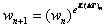.                                                      (18)

Iterative process (18) in the right part of equation has the power function with complex power coefficient. For the adequate description of such functions must be introduced the representation of the field of spiral complex numbers. If the valueis the imaginary number, then all points of iteration process (18) lay on one of exponents of circumference, which parameters determine by the meaning of.

Second type of iteration process gives the next equality: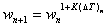.                                                 (19)

In this case we also have in the right part of equation (19) the power function with complex index of power, but the points of this iteration process lay on the exponent of circumference only    in the case of imaginary meaning ofand aspirating of its modulus to zero.